Pagini:    În jos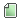Ajutor Subiect: [HackerRank] Breadth First Search: Shortest Reach  (Citit de 16717 ori) 0 Utilizatori şi 1 Vizitator pe acest subiect.
TheNechiz
De-al casei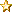Karma: 30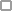Deconectat

Mesaje: 145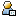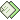« : Iulie 30, 2015, 09:16:33 »

Problem Statement

Given an undirected graph consisting of N nodes (labelled 1 to N) where a specific given node S represents the start position and an edge between any two nodes is of length 6 units in the graph.

It is required to calculate the shortest distance from start position (Node S) to all of the other nodes in the graph.

Note 1: If a node is unreachable , the distance is assumed as −1.
Note 2: The length of each edge in the graph is 6 units.

Input Format

The first line contains T, denoting the number of test cases.
First line of each test case has two integers N, denoting the number of nodes in the graph and M, denoting the number of edges in the graph.
The next M lines each consist of two space separated integers x y, where x and y denote the two node between which the edge exists.
The last line has an integer S, denoting the starting position.

Constraints
1≤T≤10
2≤N≤1000
1≤M≤N×(N−1)2
1≤x,y,S≤N

While performing the traversal, if there are edges between the same pair of nodes with different weights, the last one (most recent) is to be considered as the only edge between them.

Output Format

For each of T test cases, print a single line consisting of N−1 space separated integers, denoting the shortest distances of the N-1 nodes from starting position S.

For unreachble nodes, print −1.

Sample Input

1
4 2
1 2
1 3
1

Sample Output

6 6 -1

The graph given in the test case is shown as :S denotes the node 1 in the test case and B,C and D denote 2,3 and 4. Since S is the starting node and the shortest distances from it are (1 edge, 1 egde, Infinity) to the nodes B,C and D (2,3 and 4) respectively.

Node D is unreachable, hence -1 is printed (not Infinity).

[HackerRank] Breadth First Search: Shortest Reach

Am făcut un BFS și am memorat distanța de la S la toate nodurile într-un vector(D). Nu știu dacă am greșit la implementare, dar nu înțeleg ce vrea să zică enunțul cu observația asta:
While performing the traversal, if there are edges between the same pair of nodes with different weights, the last one (most recent) is to be considered as the only edge between them.

Cod:
# include <stdio.h>
# define NMAX 1001

int T,N,M,S,D[NMAX],Mat[NMAX][NMAX];

struct nod
{
int x,y;
struct nod *urm;
};
struct nod *P,*Q;

void Adaug( int x, int y )
{
struct nod * nou = (struct nod*)malloc(sizeof(struct nod));
nou->x = x;
nou->y = y;
nou->urm = NULL;
if( P == NULL )
P = Q = nou;
else
{
Q->urm = nou;
Q = Q->urm;
}
}

void Sterg()
{
struct nod *Z;
Z = P;
P = P->urm;
free(Z);
}

void InitD()
{
int i;
for( i = 1 ; i <= N ; ++i )
D[i] = N*N+1;
D[S] = 0;
}

void BFS()
{
int j,NodCurent,Dist;
while( P )
{
NodCurent = P->x;
Dist = P->y*6;
for( j = 1 ; j <= N ; ++j )
if( Mat[NodCurent][j] == 1 && D[j] == N*N+1 )
{
if( D[j] > Dist )
D[j] = Dist;
}
Sterg();
}
}

void Tipar()
{
int i;
for( i = 1 ; i <= N ; ++i )
{
if( i == S )
continue;
if( D[i] == N*N+1 )
D[i] = -1;
printf("%d ",D[i]);
}
putchar('\n');
}

int main()
{
int i,j,x,y;
scanf("%d",&T);
for( i = 1 ; i <= T ; ++i )
{
scanf("%d %d",&N,&M);
for( j = 1 ; j <= M ; ++j )
{
scanf("%d %d",&x,&y);
Mat[x][y] = Mat[y][x] = 1;
}
scanf("%d",&S);
InitD();
BFS();
Tipar();
}
return 0;
}

Sursa a mers pe 4 din 7 teste. Am și testele pe care a luat WA, dar ele depășesc limita de 50000 de caractere permise pe forum.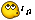Mă poate ajuta cineva ?
 « Ultima modificare: August 01, 2015, 10:55:27 de către Birisan Razvan »Memorat
 Pagini:    În sus
Schimbă forumul: